# 基于分位数回归VaR模型的股票风险实证分析Research on Stock Risk Based on Quantile Regression VaR Model

DOI: 10.12677/SA.2018.74047, PDF, HTML, XML, 下载: 818  浏览: 2,033

Abstract: VaR (VaR at Risk) is widely used to measure stock risk in recent years. In this paper, based on the historical data of nearly recent two years (Jan. 2016-Sept. 2017) of 9 stocks from the main board market, the small and medium board market and the growth enterprise board market, we compute VaR of the 9 stocks by using t-GARCH(1,1) model and Quantile-ARCH(1) model. According to the likelihood ratio test and the failure rate test, it is concluded that the Quantile-ARCH(1) model is more accurate.

1. 引言

2. 在险价值VaR

2.1. VaR的定义

VaR通常定义为：给定置信水平 $1-\alpha$ ，某一金融资产或投资组合在未来特定时间段内所面临的最大可能损失  。VaR的值非负。从统计学角度上讲，VaR的定义为：

$prob\left\{Y<-VaR\right\}=\alpha$ (1)

2.2. 基于GARCH族模型的VaR计算方法

Robert Engle在1982年提出了ARCH模型，但是为了充分描述数据的波动情况，1986年Bollerslev建立了GARCH(p,q)模型，其满足如下方程：

$\begin{array}{l}{r}_{t}=\mu +{\epsilon }_{t}\\ {\epsilon }_{t}={u}_{t}{\sigma }_{t}\\ {\sigma }_{t}^{2}=\omega +\underset{i=1}{\overset{p}{\sum }}{\alpha }_{i}{\epsilon }_{t-i}^{2}+\underset{j=1}{\overset{q}{\sum }}{\beta }_{j}{\sigma }_{t-j}^{2}\\ \left(\omega >0;{\alpha }_{i}\ge 0,i=1,2,\cdots ,p;{\beta }_{j}\ge 0,j=1,2,\cdots ,q\right)\end{array}$

3. 基于分位数回归的VaR模型

3.1. 分位数回归的基本思想

Koenker与Bassett在1978年研究了分位数回归方法，在很大程度上解决了最小二乘估计的局限性。分位数回归没有假定误差项所满足的分布形式，它在被解释变量的条件分位数对解释变量进行回归的同时考虑了误差项的异方差性  。

${Q}_{\alpha }\left(y|x\right)=\underset{y\in {R}^{1}}{\mathrm{inf}}\left\{y:{F}_{y|x}\left(y|x\right)\ge \alpha \right\}$

${F}_{y|x}$ 表示已知x的条件下y的分布函数， $\alpha$ 表示预先设定的分位点。线性分位数回归的 $\alpha$ 条件分位数函数为：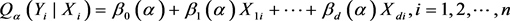$\mathrm{min}\underset{i=1}{\overset{n}{\sum }}{\rho }_{\alpha }\left({Y}_{i}-\underset{j=1}{\overset{d}{\sum }}{\beta }_{j}\left(\alpha \right){X}_{i}\right)=\mathrm{min}\left[\alpha \underset{y\ge {x}^{\prime }\beta \left(\alpha \right)}{\sum }|{Y}_{i}-\underset{j=1}{\overset{d}{\sum }}{\beta }_{j}\left(\alpha \right){X}_{i}|+\left(1-\alpha \right)\underset{y<{x}^{\prime }\beta \left(\alpha \right)}{\sum }|{Y}_{i}-\underset{j=1}{\overset{d}{\sum }}{\beta }_{j}\left(\alpha \right){X}_{i}|\right]$

3.2. 分位数回归VaR模型

Koenker和Zhao提出了Quantile-ARCH模型  ，其回归模型如下：

${r}_{t}={\beta }^{\text{T}}{x}_{t}+{\epsilon }_{t}$ (2)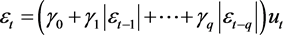(3)

${F}_{t-1}$ 已知的情况下， ${r}_{t}$ 的条件 $\alpha$ 分位数为：

${Q}_{\alpha }\left({r}_{t}|{F}_{t-1}\right)={\beta }^{\text{T}}{x}_{t}+{Q}_{\alpha }\left({\epsilon }_{t}|{F}_{t-1}\right)$ (4)

${Q}_{\alpha }\left({\epsilon }_{t}|{F}_{t-1}\right)={\gamma }^{\text{T}}\left(\alpha \right){W}_{t}={\left({\gamma }_{0}{Q}_{\alpha }\left({u}_{t}\right),{\gamma }_{1}{Q}_{\alpha }\left({u}_{t}\right),\cdots ,{\gamma }_{q}{Q}_{\alpha }\left({u}_{t}\right)\right)}^{\text{T}}{W}_{t}$ (5)

$\text{prob}\left({r}_{t}<-\text{VaR}|{F}_{t-1}\right)=\alpha$ (6)

${\text{VaR}}_{t}\left(\alpha \right)=-{Q}_{\alpha }\left({r}_{t}|{F}_{t-1}\right)=-{\beta }^{\text{T}}{x}_{t}-{Q}_{\alpha }\left({\epsilon }_{t}|{F}_{t-1}\right)=-{\beta }^{\text{T}}{x}_{t}-{\gamma }^{\text{T}}\left(\alpha \right){W}_{t}$ (7)

4. 实证分析

4.1. 数据选取

4.2. 基本统计特征分析

$\text{JB}=\frac{n}{6}\left[{S}^{2}+\frac{{\left(K-3\right)}^{2}}{4}\right]$ (8)

4.3. 平稳性检验

4.4. 条件异方差检验Table 2. ADF, PP, KPSS Test

4.5. 基于GARCH族类模型的VaR计算

t-GARCH(1,1)模型：

$\begin{array}{l}{r}_{t}={\mu }_{t}+{\epsilon }_{t}\\ {\sigma }_{t}^{2}=\omega +\alpha {\epsilon }_{t-1}^{2}+\beta {\sigma }_{t-1}^{2}\end{array}$Table 5. t-GARCH(1,1) ModelTable 6. Caculation of VaR by t-GARCH(1,1) Model

4.6. 基于分位数回归方法的VaR的计算

$\begin{array}{l}{r}_{t}={\mu }_{t}+{\epsilon }_{t}\\ {\epsilon }_{t}=\left({\gamma }_{0}+{\gamma }_{1}|{\epsilon }_{t-1}|\right){u}_{t}\end{array}$

4.7. 两种VaR计算模型的比较Table 7. Caculation of VaR by Quantile-ARCH(1) Model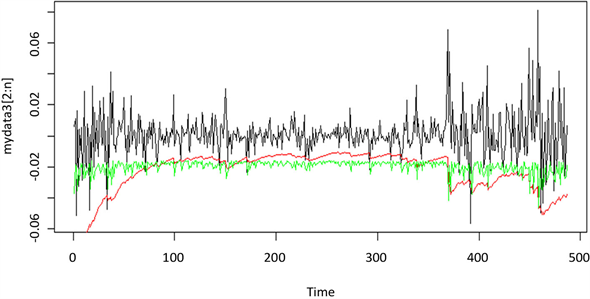Figure 1. Yield curve & t-GARCH(1,1) VaR & Quantile-ARCH(1) VaR

$\text{LR}=2\mathrm{ln}\left[{\left(1-N/n\right)}^{n-N}{\left(N/n\right)}^{N}\right]-2\mathrm{ln}\left[{\left(1-{p}^{*}\right)}^{n-N}{\left({p}^{*}\right)}^{N}\right]\sim {\chi }^{2}\left(1\right)$Table 8. Comparison of two models

5. 结论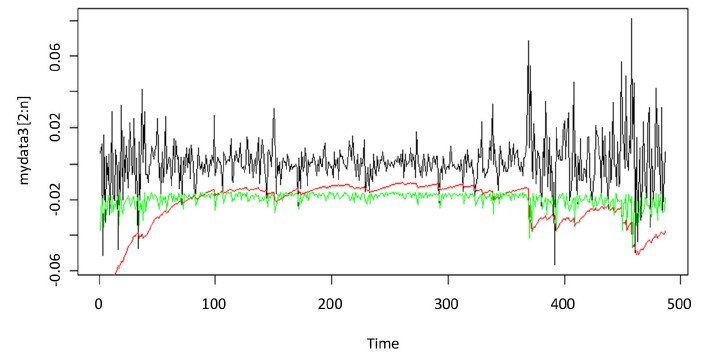Figure 1. Yield curve & t-GARCH(1,1) VaR & Quantile-ARCH(1) VaR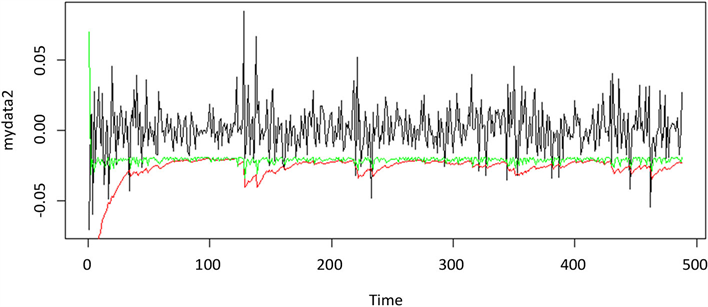Figure 2. Yield curve & t-GARCH(1,1) VaR & Quantile-ARCH(1) VaR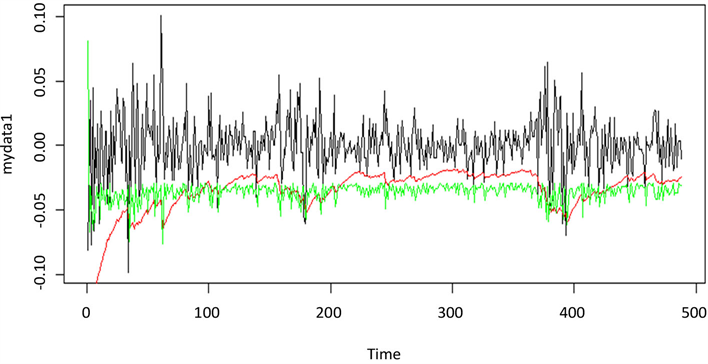Figure 3. Yield curve & t-GARCH(1,1) VaR & Quantile-ARCH(1) VaR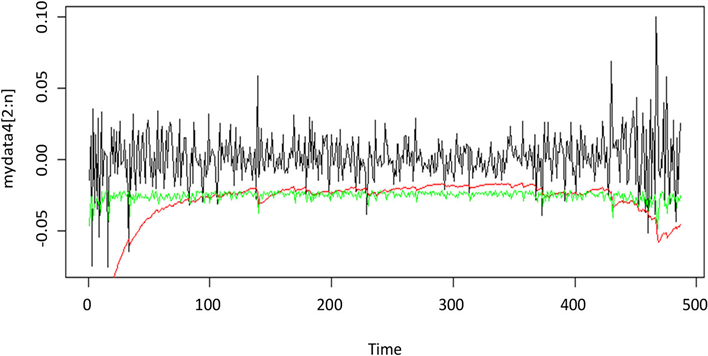Figure 4. Yield curve & t-GARCH(1,1) VaR & Quantile-ARCH(1) VaRFigure 5. Yield curve & t-GARCH(1,1) VaR & Quantile-ARCH(1) VaR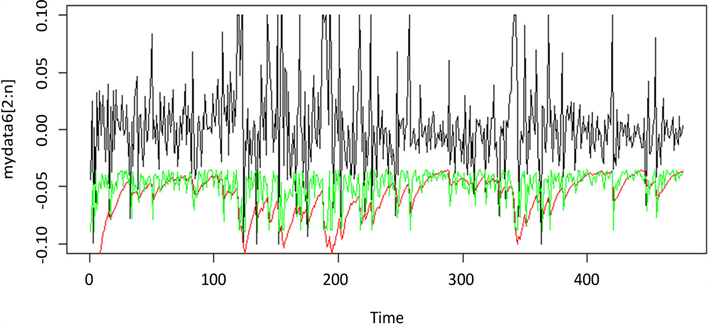Figure 6. Yield curve & t-GARCH(1,1) VaR & Quantile-ARCH(1) VaR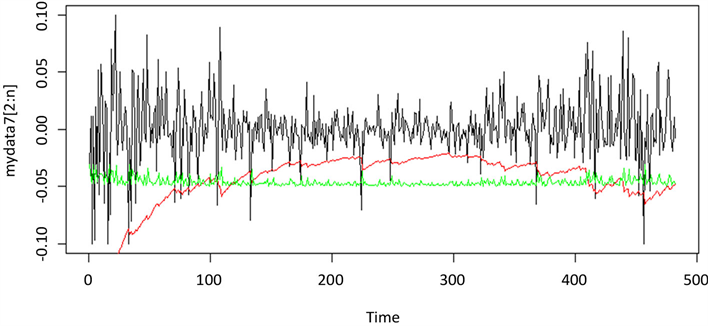Figure 7. Yield curve & t-GARCH(1,1) VaR & Quantile-ARCH(1) VaR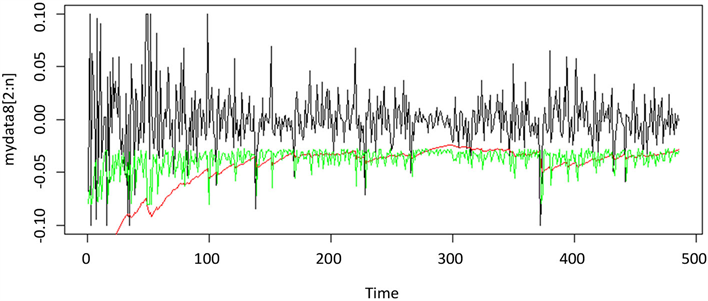Figure 8. Yield curve & t-GARCH(1,1) VaR & Quantile-ARCH(1) VaR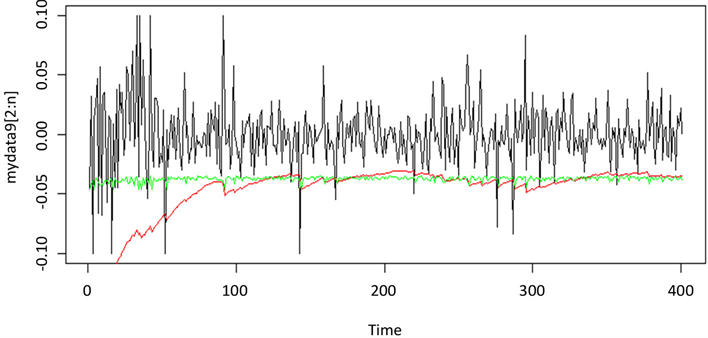Figure 9. Yield curve & t-GARCH(1,1) VaR & Quantile-ARCH(1) VaR

  叶五一. VaR与CVaR的估计方法以及在风险管理中的应用[D]: [博士学位论文]. 合肥: 中国科学技术大学, 2006.  龚锐. 在险价值(VaR)方法在中国金融市场风险度量中的应用[D]: [硕士学位论文]. 重庆: 重庆大学, 2005.  刘庆富, 仲伟俊, 梅姝娥. 基于VaR-GARCH 模型族的我国期铜市场风险度量研究[J]. 系统工程学报, 2006(21): 429-433.  张珏. 基于分位数回归模型的证券市场风险研究[J]. 统计与决策, 2011(9): 61-63.  解其昌. 分位数回归方法及其在金融市场风险价值预测中的应用[D]: [博士学位论文]. 成都: 西南财经大学, 2012.  涂振兴. 基于分位数回归VaR模型对中国证券市场的研究[D]: [硕士学位论文]. 成都: 西南财经大学, 2016.  张成. 基于分位数回归的金融风险管理[D]: [硕士学位论文]. 成都: 西南交通大学, 2016.  Jonathan D. Cryer, Kung-Sik Chan. 时间序列分析及应用R语言[M]. 北京: 机械工业出版社, 2011.  王星, 褚挺进. 非参数统计(第2版)[M]. 北京: 清华大学出版社, 2009.  叶五一, 缪柏其, 吴振翔. 基于Bootstrap方法的VaR计算[J]. 系统工程学报, 2004, 19(5): 528-531.  杨夫立. 证券投资基金市场风险度量研究-基于GARCH模型、VaR及CVaR方法与Copula函数的视角[D]: [博士学位论文]. 天津: 南开大学, 2012.